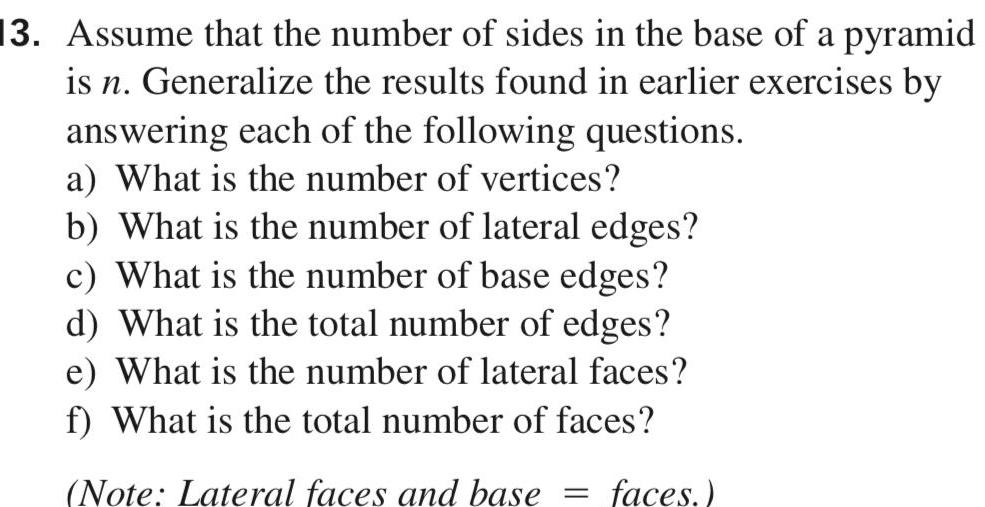Question:

# 13 Assume that the number of sides in the base of a pyramid

Last updated: 7/26/202313 Assume that the number of sides in the base of a pyramid is n Generalize the results found in earlier exercises by answering each of the following questions a What is the number of vertices b What is the number of lateral edges c What is the number of base edges d What is the total number of edges e What is the number of lateral faces f What is the total number of faces Note Lateral faces and base faces